• 今天，我们一起来学习基本绘图常用函数。在MATLAB中绘图首先需要设定x的范围，一般使用冒号来创建向量。例如 x = 0:pi/100:pi; &x 为0到pi之间以pi/100为步长的向量。(1) plot()二维图形的绘制要求：x和y需要有...点击上方蓝字  关注我们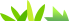MATLAB有很强的绘图功能，我们可以通过简单的代码画出一些直观而漂亮的代码。今天，我们一起来学习基本绘图常用函数。
在MATLAB中绘图首先需要设定x的范围，一般使用冒号来创建向量。例如
 x = 0:pi/100:pi;
 &x 为0到pi之间以pi/100为步长的向量。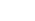(1) plot()二维图形的绘制
要求：x和y需要有相同长度的向量
调用形式：
plot(x)  :绘制出以x为纵坐标值的折线图，横轴值默认为1、2、3……。
plot(x,y): 绘制出以x向量为自变量的y函数在给出x的范围内的图像。
plot(y):  在给定的x范围内绘制出函数y的图像。
plot(x1,y1,……xn,yn):绘制多个X、Y对组的图，注意所有线条都使用相同的坐标区。(x1,x2,x3……xn都应相同)
plot(x,y'……') 此类形式方便对线形颜色等属性的修改。
>> x = 0:pi/100:2*pi;>> y = sin(x);>> y1 = cos(x);>> plot(x);>> plot(x,y);>> plot(x,y,x,y1);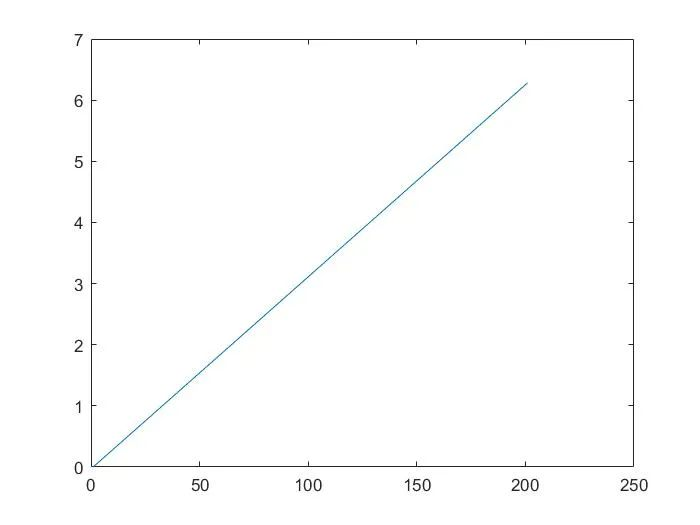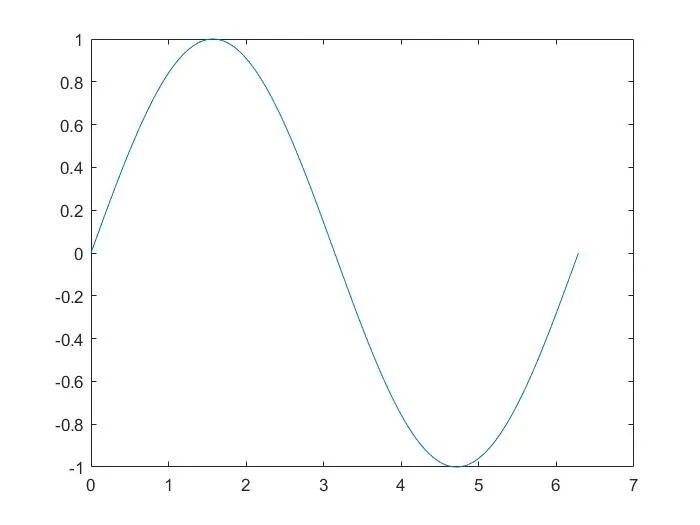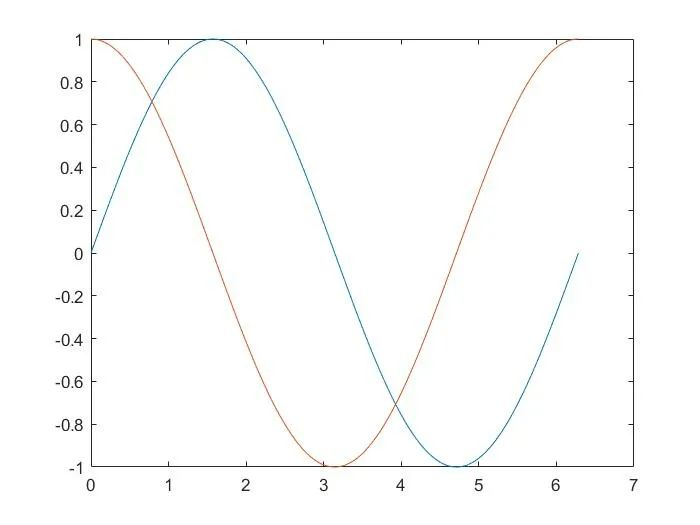(2) fplot()符号函数的绘制
函数fplot可以根据函数的表达式自动调整自变量的范围，无须给函数赋值，直接生成能反应函数变化规律的图形，
调用格式:
fplot(y) : 在默认区间[-5 5](对于x)绘制由函数y = f(x)定义的曲线。
fplot(y,xinterval):将在指定区间绘图。将区间指定为[xmin xmax]形式的二元素向量。
fplot(funx,funy):在默认区间[-5 5](对于t)绘制由x = funx(t)和y = funy(t)定义的曲线。
fplot(funx,funy,interval):将在指定区间绘图。将区间指定为[tmin tmax]形式的二元素向量。
fplot(  ,linespace)   linespace 为线形，次函数可以修改所绘制图像曲线的线形。
>> fplot(@(x) sin(x))
%绘制匿名函数，使用句柄。>> fplot(@(t)t.*sin(t),@(t)t.*cos(t))
%绘制参数方程，x =t*sin(t);y =t*cos(t);&其它例子大致相同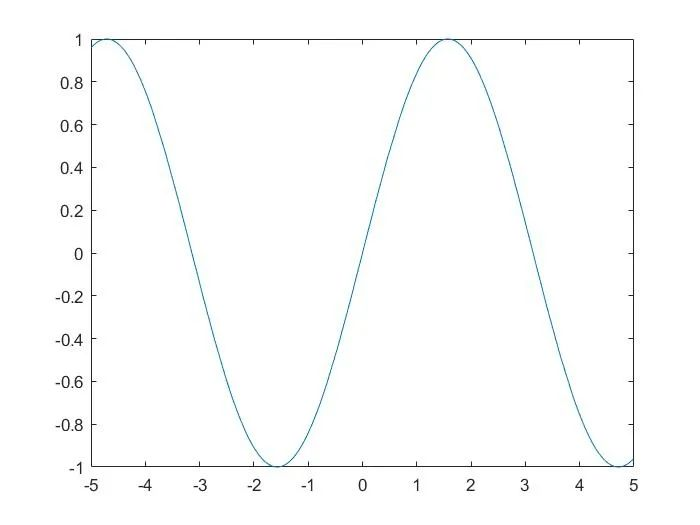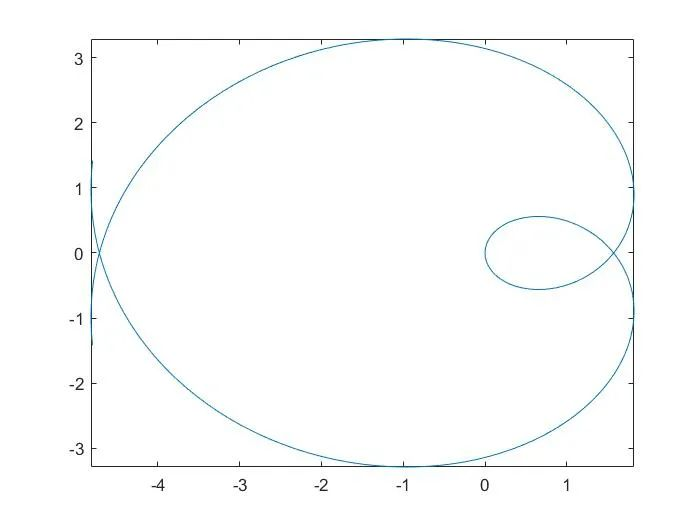注意该fplot函数中的y以及funx,funy所代表的函数，它们一般是以句柄的形式给出。
关于MATLAB中句柄的建立以及使用请参考：
(3)plotyy()双Y轴图形绘制
该函数可以在一个图中创建有两个Y轴的图形。
调用格式：
plotyy(x1,y1,x2,y2):  绘制Y1对X1的图，在左侧显示y轴标签，并同时绘制Y2对X2的图，在右侧显示y轴标签。
plotyy(x1,y1,x2,y2,function):使用指定的绘图函数生成图形。
常见的function函数有plot、semilogx(半对数图中按照x轴的对数刻度绘制数据。)、semilogy(半对数图中使用y轴的对数刻度绘制数据。)、loglog(双对数图)、stem(绘制离散序列数据)
详细参见文末链接。
plotyy(x1,y1,x2,y2,'function1','function2'):使用function1(X1,Y1)绘制左轴的数据，使用function2(X2,Y2)绘制右轴的数据。
>> x = 0:pi/100:2*pi;>> y1 = sin(x);>> y2 = cos(x);>> plotyy(x,y1,x,y2)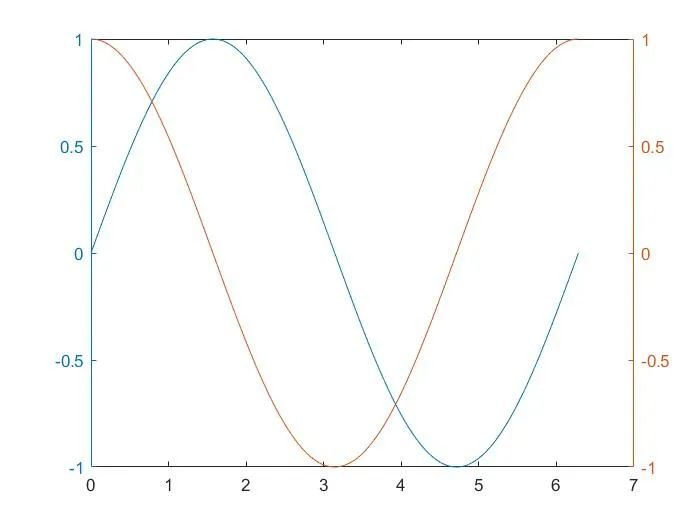>> x = [0,10^6,7]>> y3 = x;>> y4 = exp(x);>> plotyy(x,y3,x,y4,'semilogx')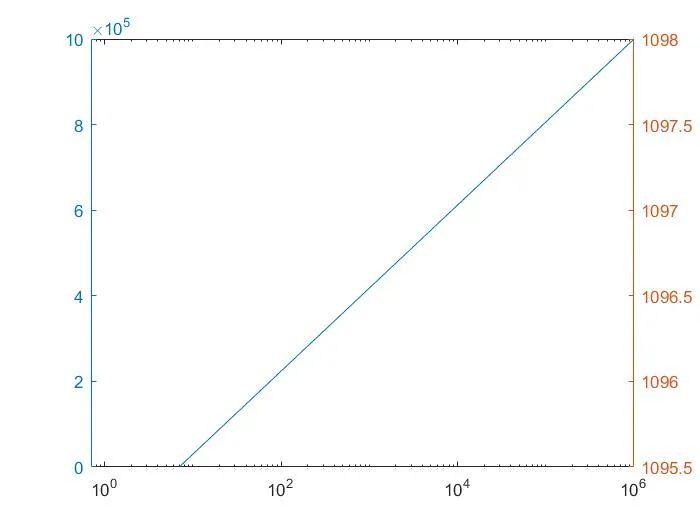(4) ezplot() 符号函数的绘制
ezplot()函数与fplot函数的功能基本相似，可以方便地绘制表达式或函数的图形。与fplot函数不同的是函数的表达式显示在图形的上方，同时对坐标轴可以不加任何限制作图。
调用格式：
ezplot(fun):  绘制表达式fun(x)在默认定义域 -2π <x< 2π 上的图形，其中fun(x)仅是x的显函数。
ezplot(fun,[xmin,xmax]):绘制fun(x)在以下域上的图形：xmin<x<xmax。
对于隐函数，fun2(x,y)：
ezplot(fun2) 在默认域 -2π x < 2π 和 -2π y < 2π 中绘制 fun2(x,y) = 0。
ezplot(fun2,[xymin,xymax]) 在 xymin x xymax 和 xymin y xymax 域中绘制 fun2(x,y) = 0。
ezplot(fun2,[xmin,xmax,ymin,ymax]) 在 xmin x xmax 和 ymin y ymax 域中绘制 fun2(x,y) = 0。
>> subplot(221)>> ezplot('x^2+sin(x)');>> subplot(222)>> ezplot('x^2+sin(x)',[-2,8])>> subplot(223)    %subplot()函数可以在一张图幅上画出多个图像，如下图所示。>> subplot(2,2,[3,4])>> ezplot('x^2+sin(x)',[-2,5,-1,20])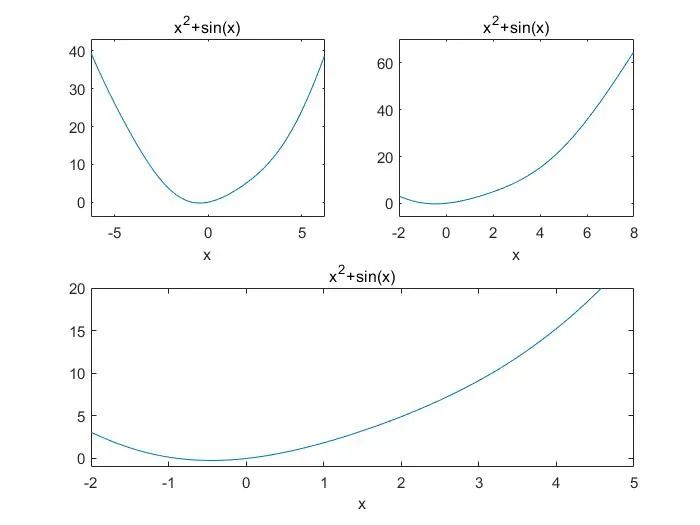END
对于半对数图，双对数图参考以下链接：
半对数图—MATLAB semilogx:
半对数图—MATLAB semilogy:
双对数刻度图—MATLAB loglog:
绘制离散序列数据—MATLAB stem:
本文参考：
清华大学出版社《MATLAB基础与可视化编程》
MATLAB MathWorks官网
本文仅供学习交流所用，由于能力不足，编写不当之处望批评指正。
关于MATLAB的学习：
大家可以关注我们的知乎专栏——数据可视化和数据分析中matlab的使用：
https://zhuanlan.zhihu.com/c_1131568134137692160
欢迎大家加入我们的MATLAB学习交流群：
953314432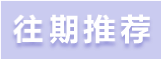MATLAB界面介绍及学习方法(附有视频讲解)
数学建模与MATLAB–整数规划(附有代码讲解)
数学建模与MATLAB--非线性规划
数学建模与MATLAB——动态规划扫码关注我们
了解更多精彩
出品：Asoul水云天课堂工作室


展开全文• 1、已经有多组数据的情况下，绘制x-y折线图。 #!/usr/bin/env python3 # -*- coding: utf-8 -*- """ Created on Mon Apr 1 13:38:37 2019 绘制折线图 @author: youxinlin """ import numpy as np import ...
1、已经有多组数据的情况下，绘制x-y折线图。
#!/usr/bin/env python3
# -*- coding: utf-8 -*-
"""
Created on Mon Apr  1 13:38:37 2019
绘制折线图

@author: youxinlin
"""

import numpy as np
import matplotlib as mpl
import matplotlib.pyplot as plt
plt.title('elapsed time')

x_axis = range(5)
y_data1 =[0.05188298225402832,0.045903921127319336,0.03152298927307129,0.026485919952392578,0.027455806732177734]
y_data2=[1.4355370998382568, 1.4685750007629395, 1.4546618461608887, 1.4545753002166748]

plt.plot(x_axis, y_data1, color='red', label='my algorithm')
plt.plot(x_axis, y_data2, color='green', label='other')
plt.legend() # 显示图例
plt.xlabel('x label')
plt.ylabel('time cost')
plt.show()

用你的数据，替换x和y_data。
2、根据表达式绘制函数图像。
import numpy as np
from matplotlib import pyplot as plt
import math
theta = np.linspace(0.01,np.pi/2-0.1)
l = 2*np.tan(theta)

r1 = ( (np.cos(theta)-1.33*np.sqrt(1- (1/1.33*np.sin(theta))**2)) / (np.cos(theta)+1.33*np.sqrt(1- (1/1.33*np.sin(theta))**2)))**2
r2 = ( np.sqrt(1- (1/1.33*np.sin(theta))**2-1.33*np.cos(theta)) /  np.sqrt(1- (1/1.33*np.sin(theta))**2+1.33*np.cos(theta)))**2

for i in range(50):
if math.isnan( r2[i] ):
r2[i]=0

Rr = 0.5*(r1+r2)

plt.figure(1)
plt.figure(figsize=(9, 6))

plt.xlabel(r"$l/m$",fontdict={'weight': 'normal', 'size': 20})
plt.ylabel(r"$\mathregular{R_r}$",fontdict={'weight': 'normal', 'size': 20})

#plt.ylim(-0.1, 1)
#plt.title("Reflectance")
#plt.annotate('max', xy=(0.22, 0.9), xytext=(0.22, 0.5),arrowprops=dict(facecolor='black'))

plt.plot(theta,Rr) #打印Rr和theta的关系
plt.plot(l,Rr) #打印Rr和L的关系

plt.show()

上面这个例子是自己科研过程中用到的；Rr是关于theta的直接函数，theta又是关于l的函数，所以Rr和l有关系，懒得求公式的话，直接plot出Rr和l，也可以“隔山打牛”，很方便地得到它们直接的函数图像。
这个例子中，如果要在label中打出theta这个特殊符号用到的方法是：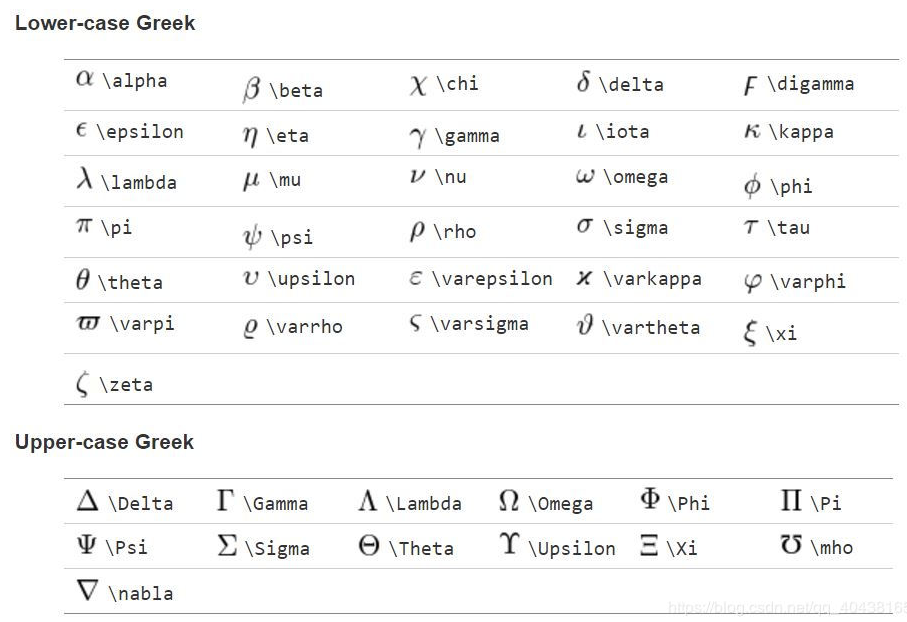3、绘制不同颜色、形态的折线图
在属性值先写颜色，后写形状如：r-(红色曲线),b–(蓝色短横线)等。例如：
plt.figure(1)
plt.figure(figsize=(13,6))
plt.plot(x_axis, Fbeita_list, 'r-o', label=r"$\mathregular{F_\beta}$")
plt.plot(x_axis, y_precise, 'b--^', label='P')
plt.plot(x_axis, y_recall, 'b--s', label='R')
字符描述'-'实线样式'--'短横线样式'-.'点划线样式':'虚线样式'.'点标记','像素标记'o'圆标记'v'倒三角标记'^'正三角标记'&lt;'左三角标记'&gt;'右三角标记'1'下箭头标记'2'上箭头标记'3'左箭头标记'4'右箭头标记's'正方形标记'p'五边形标记'*'星形标记'h'六边形标记 1'H'六边形标记 2'+'加号标记'x'X 标记'D'菱形标记'd'窄菱形标记'&#124;'竖直线标记'_'水平线标记
字符颜色'b'蓝色'g'绿色'r'红色'c'青色'm'品红色'y'黄色'k'黑色'w'白色
给字母添加上下标：https://blog.csdn.net/weixin_38314865/article/details/89392911
如果要逆序坐标轴，加上：
plt.gca().invert_xaxis()   
展开全文python plt
• Tableau使用心得第二期：折线图、柱状图和字符串函数Tableau使用心得折线图柱状图函数介绍字符串函数总结 Tableau使用心得 上一期简单介绍了Tableau的使用步骤，主要为导入数据、选择维度和图表呈现三大步。其次罗列...


Tableau使用心得第二期：折线图、柱状图和字符串函数
Tableau使用心得折线图柱状图函数介绍字符串函数
总结

Tableau使用心得
上一期简单介绍了Tableau的使用步骤，主要为导入数据、选择维度和图表呈现三大步。其次罗列了所有数字函数的用法和表示，熟悉了的用户可以自己试着增加含有数字函数的维度。这一期我们会着重介绍折线图和柱状图的画法，此两类图表可以说是基础图表中最为常见的，而后我们会在此罗列出所有字符串函数的用法和相应表示。
折线图
折线图是以线性趋势的形式绘制工作表中的行或列数据的图表的一种展示形式。折线图可以显示随时间、项目、类别等各类维度变化的连续数据，因此非常适用于显示在相等类别间隔下数据的趋势。在折线图中，类别数据沿水平轴均匀分布，数值数据沿垂直轴均匀分布。
在Tableau中，绘制折线图也相当简单，在左边的 Marks 处选择 Line 选项即可。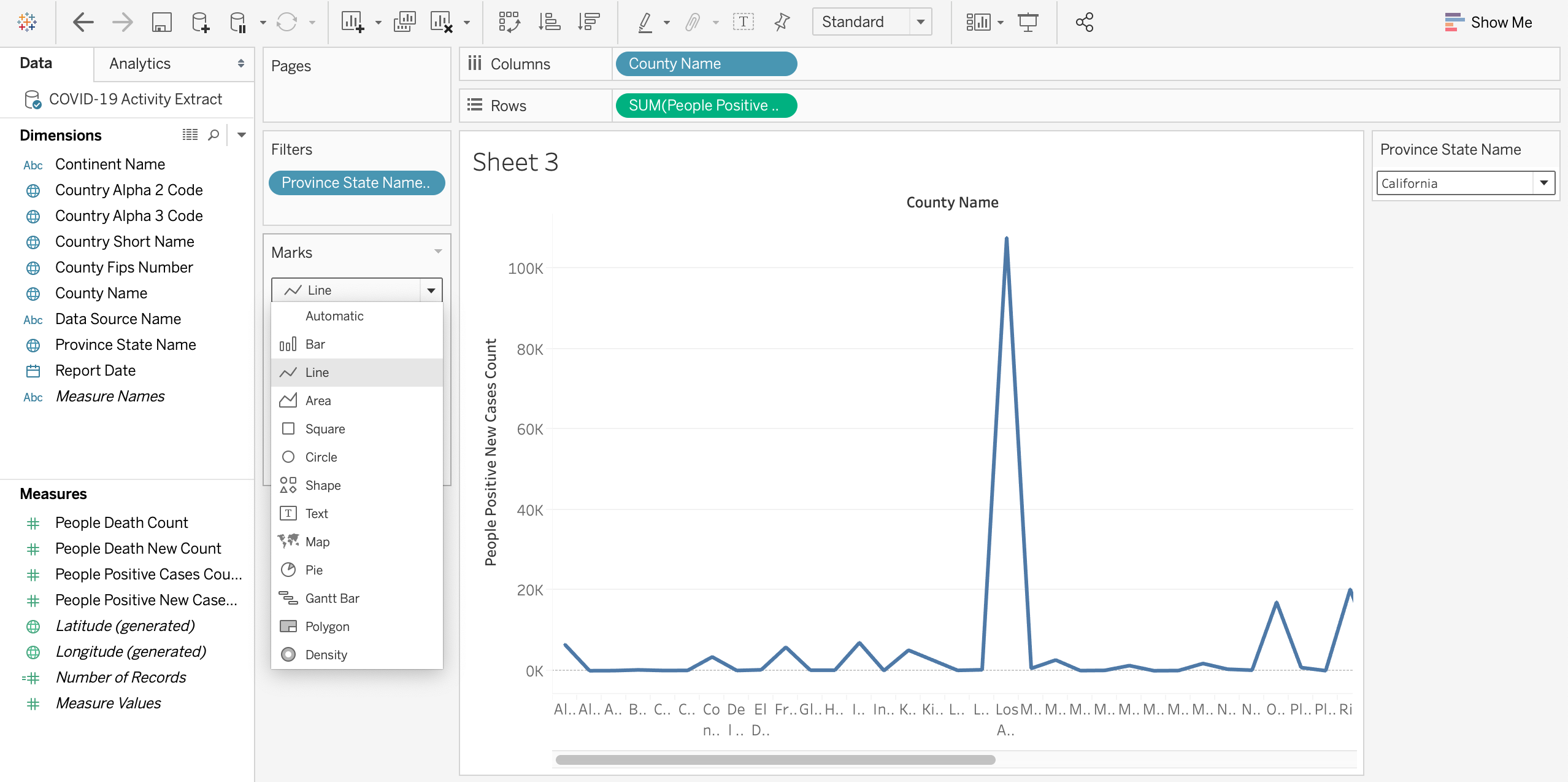在右下角的 Path 还可以选择折线的类型 Line Type，下图显示的为阶梯式折线图。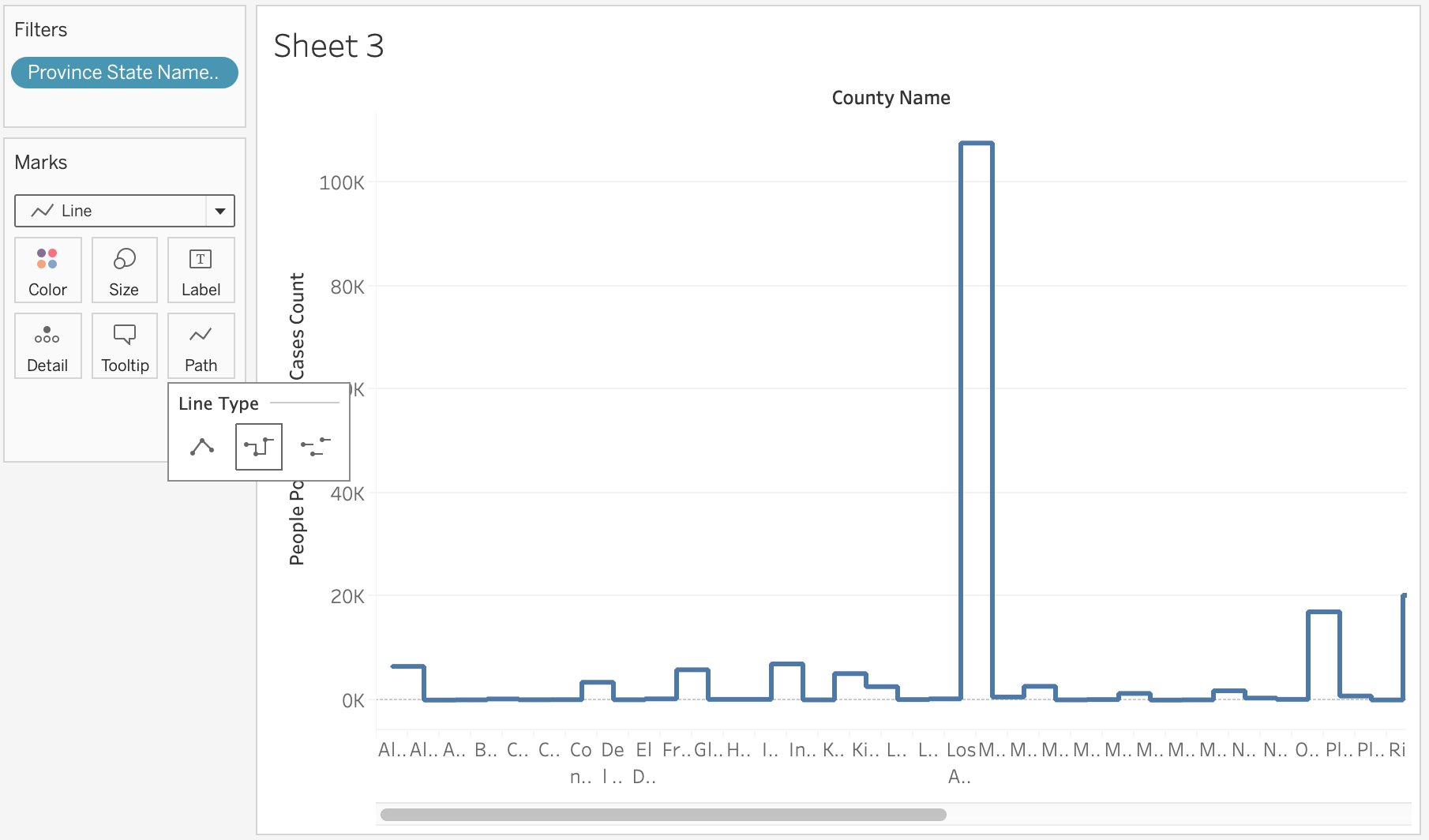如果选择Label，还可以显示折线处的各类数值。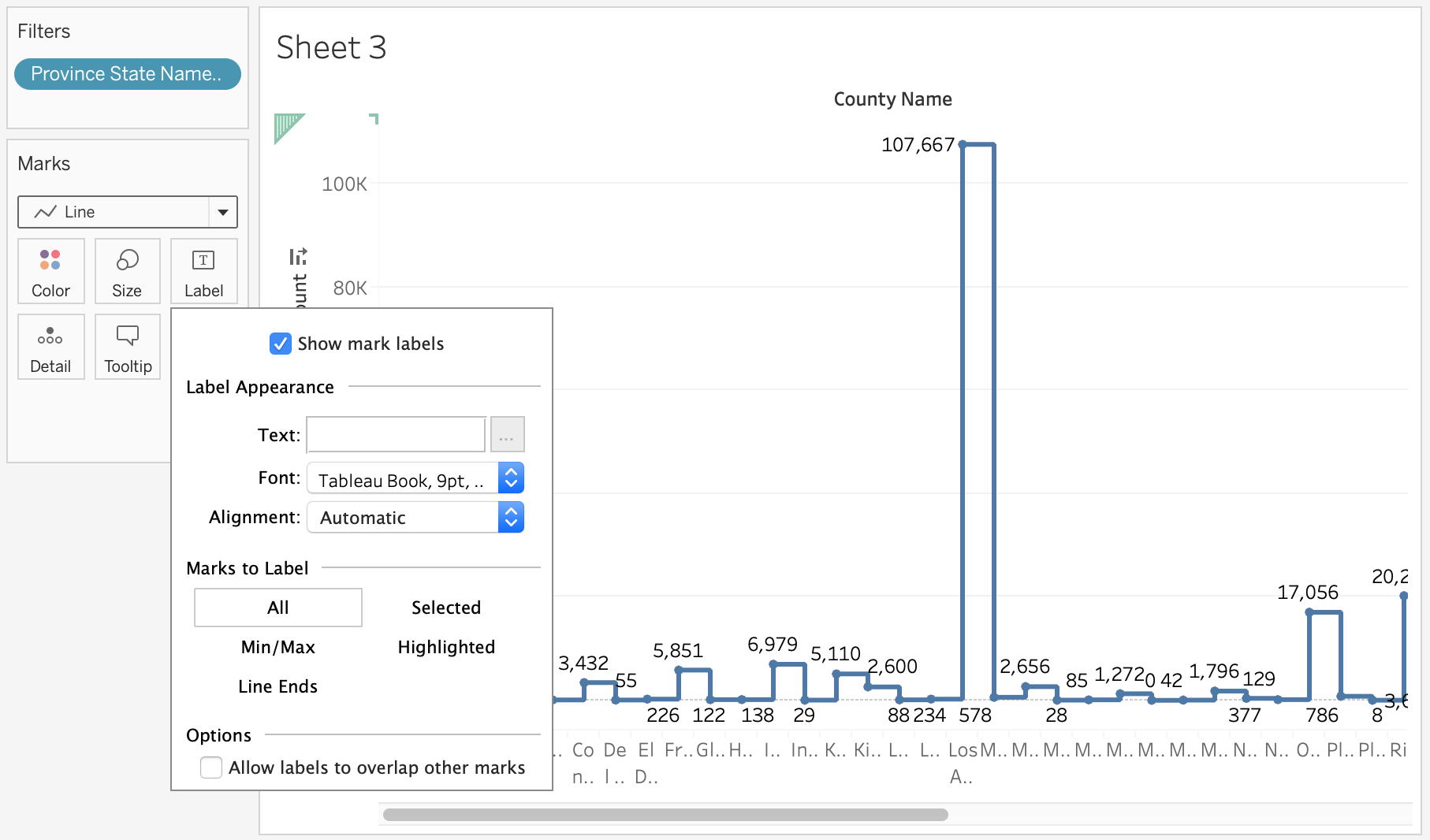同样，颜色 Color 和大小 Size 可以调整折线图中线的粗细还有线的颜色，这类调整在所有图形中都可以应用，这里就不多做展示了。
柱状图
柱形图，又称直方图、长条图、柱状图、条状图、棒形图，是一种以长方形的长度为变量的统计图表。柱形图用来比较两个或以上的价值，只有一个变量，通常利用于较小的数据集分析。在统计学中，直方图是一种对数据分布情况的图形表示，是一种二维统计图表，它的两个坐标分别是统计样本和该样本对应的某个属性的度量。
同样，和折线图类似，在 Marks 选框中选择 Bar，即可绘制柱状图。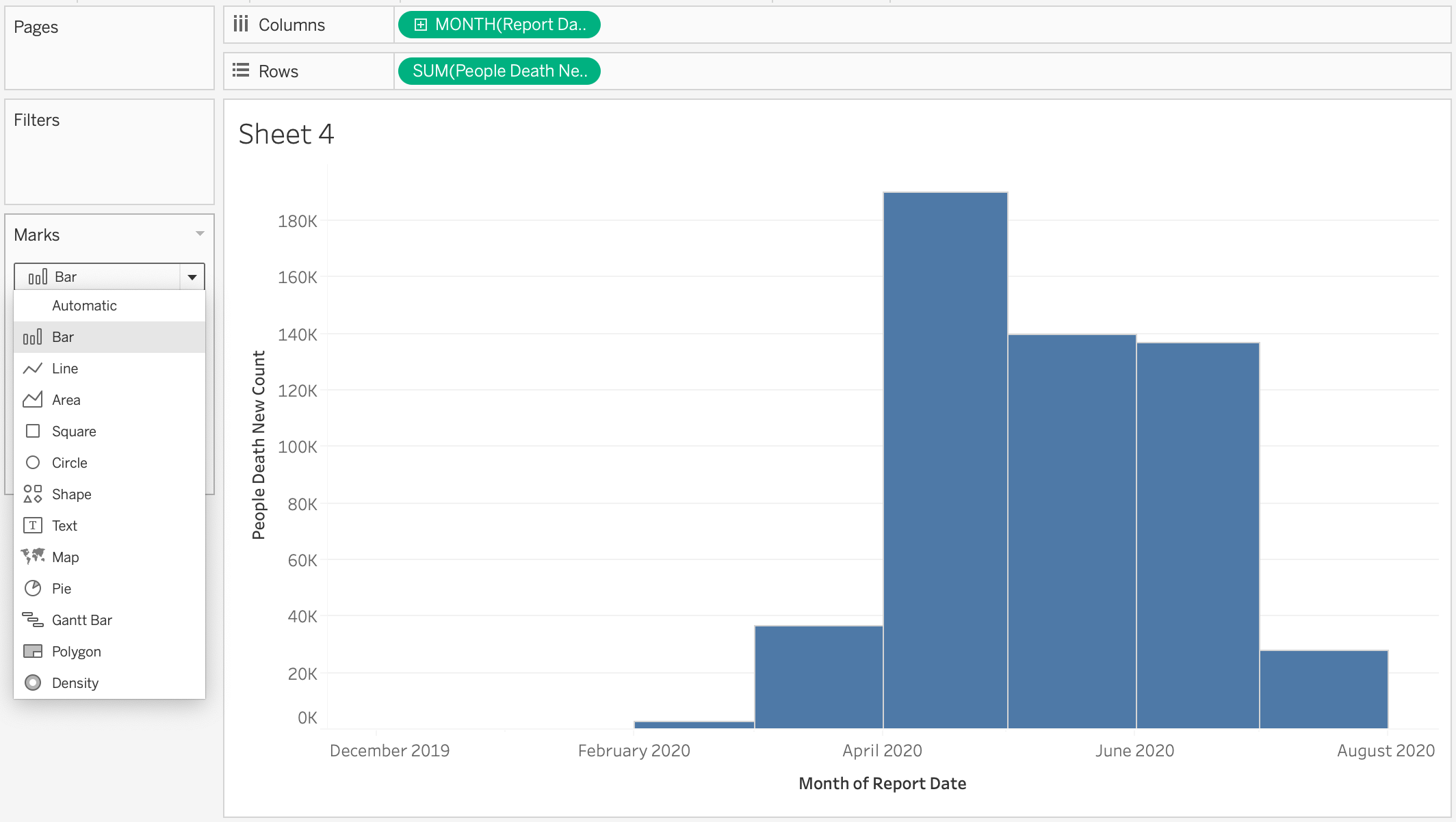一些在ggplot中能做到的功能，Tableau也可以轻而易举地做到，比如带有第三类参数的堆叠柱状图：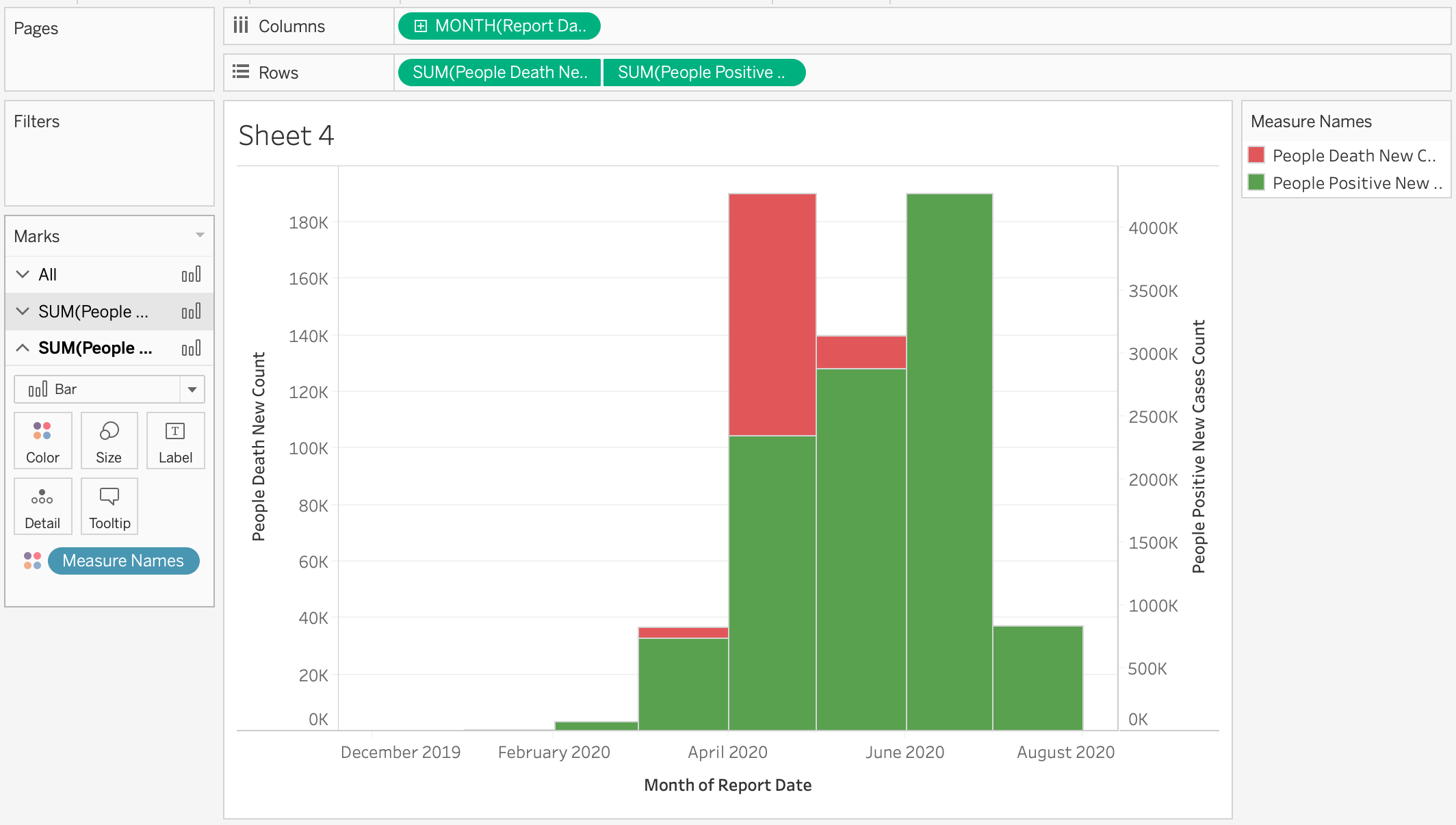也可以通过 Size 调整柱状图大小：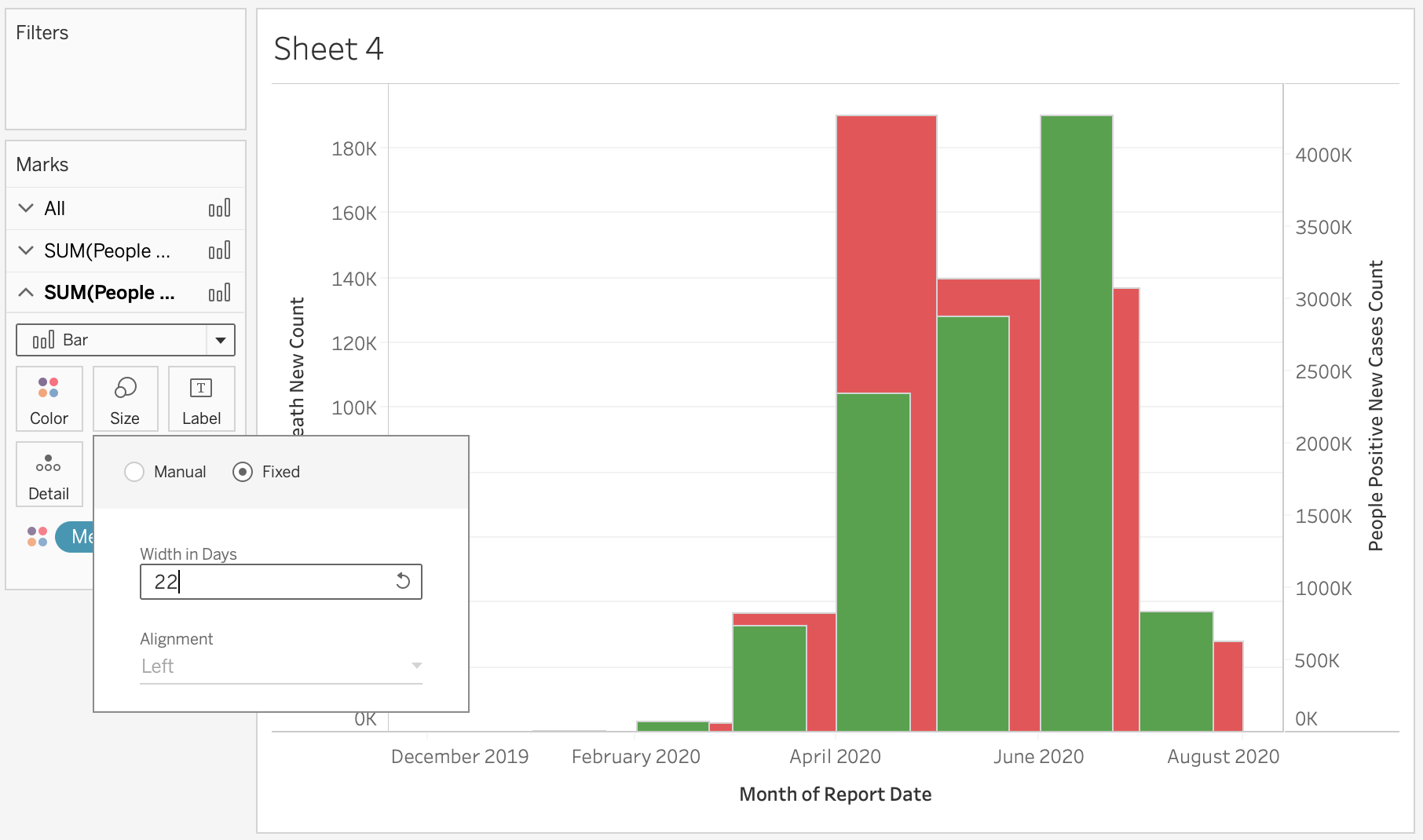函数介绍
上一章我们主要介绍了数字函数，这一节我们将列举所有字符串函数和它们的用途。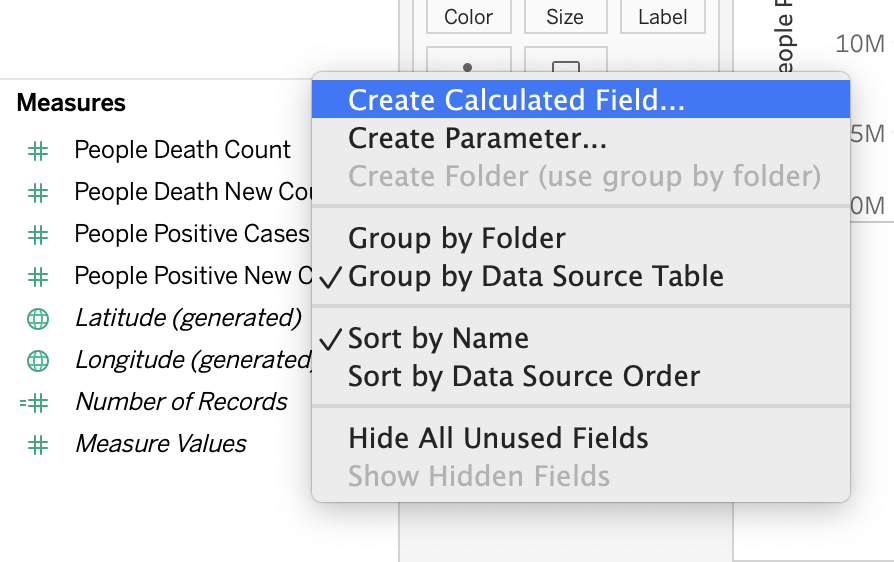字符串函数
意义函数例子ASCII码ASCII(string)ASCII(‘A’) = 65反ASCII码CHAR(number)CHAR(65) = ‘A’是否包含字符串CONTAINS(string, substring)CONTAINS(“Calculation”, “alcu”) = true是否指定字符串结尾ENDSWITH(string, substring)ENDSWITH(“Tableau”, “leau”) = true索引位置FIND(string, substring, [start])FIND(“Calculation”, “a”, 2) = 2索引n位置FINDNTH(string, substring, occurrence)FINDNTH(“Calculation”, “a”, 2) = 7左侧字符LEFT(string, number)LEFT(“Matador”, 4) = “Mata”字符串长度LEN(string)LEN(“Matador”) = 7小写LOWER(string)LOWER(“ProductVersion”) = “productversion”移除前空格LTRIM(string)LTRIM(" Matador ") = "Matador "较大值MAX(a, b)MAX (“Apple”,“Banana”) = “Banana”索引字符串MID(string, start, [length])FLOOR(3.1415) = 3较小值MIN(a, b)MIN (“Apple”,“Banana”) = “Apple”替换REPLACE(string, substring, replacement)REPLACE(“Version8.5”, “8.5”, “9.0”) = “Version9.0”右侧字符RIGHT(string, number)RIGHT(“Calculation”, 4) = “tion”移除后空格RTRIM(string)RTRIM(" Calculation “) = " Calculation”空格字符串SPACE(number)SPACE(1) = " "子字符串SPLIT(string, delimiter, token number)SPLIT (‘a-b-c-d’, ‘-‘, 2) = ‘b’是否开头STARTSWITH(string, substring)STARTSWITH(“Joker”, “Jo”) = true移除前后空格TRIM(string)TRIM(" Calculation ") = “Calculation”大写UPPER(string)UPPER(“Calculation”) = “CALCULATION”
更多介绍详见 字符串函数
总结
本期主要介绍了折线图和柱状图的绘制方法，其实大致步骤和诀窍都差不多，综合来说在Tableau中绘制任何图形都离不开“简单”二字，这也是这个软件在用户中如此火热的原油、紧接着，本章又介绍了各类字符串函数和它们的用途，函数的通用使这款软件可以面向编程零基础的用户，以最简单快速的方式，呈现简约有效的数据处理。 之后的几期会把Tableau中常用的函数依依列举完成，最后一期会介绍一些Tableau界面设计，毕竟对于如此便捷的数据处理软件，界面美化也是它的一个卖点之一。 感谢您的关注，如果喜欢请点赞收藏本文，谢谢！
展开全文数据可视化 数据分析
• 原博文2018-08-02 15:44 −折线图 也称为趋势图，它使用直线... 折线图绘制函数： plot(x,y,style,color,linewidth) &n...相关推荐2019-12-18 17:55 −以代码一开头：代码一：import matplotlib.pyplot as pltimport...
原博文
2018-08-02 15:44 −
折线图 也称为趋势图，它使用直线线段将个数据点连接起来而组成的图形，以折线方式显示数据的变化趋势。 折线图绘制函数： plot(x,y,style,color,linewidth) &n...
相关推荐
2019-12-18 17:55 −
以代码一开头：
代码一：import matplotlib.pyplot as plt
import numpy as np
import pandas as pd
plt.rcParams['font.sans-serif']=['SimHei'] #用来正常显示中文标签
plt.rcParam...
2019-12-18 16:45 −
[TOC]
# 1.pyplot基础语法
（1）创建画布
figure()创建一个空白画布，可以指定画布的大小figsize和设置分辨率dpi
python
plt.figure() plt.figure(figsize=(8,6),dpi=80) #（8，6)代表图像的长和宽单位是英寸，dpi...
2019-12-04 09:20 −
来自：https://www.cnblogs.com/dudududu/p/9149762.html Python有很多可视化工具，本篇只介绍Matplotlib。 Matplotlib是一种2D的绘图库，它可以支持硬拷贝和跨系统的交互，它可以在Python脚本、IPython的交互环境下、Web应用...
2019-11-11 16:40 −
eval() 函数，用来执行一个字符串表达式，并返回表达式的值。
score = eval(input("enter the score: "))
第三章 数据获取
抓取： requests库、第...
2019-12-14 11:06 −
## 数据分析07 /matplotlib绘图 [TOC] ### 1. 绘制线性图：plt.plot() - 绘制单条线形图 python import matplotlib.pyplot as plt import numpy as np x = [1,2,3,4,5] y = [...
2019-12-18 17:16 −
1．椭圆型布纹图案
先在HTML页面中设置一个画布。

worksheet = ...
2019-12-13 16:57 −
一、属性
Properties属性Description简介
text-decoration
复合属性。检索或设置对象中的文本的装饰。
text-decoration-line
检索或设置对象中的文本装饰线条的位置。
text-decoration-color
检索或设置对象中...
2019-12-06 17:40 −
#1. R 中图形的概念
>####在 R 中图，就像 photoshop 中的图层一样，每一个元素都是层层向上延展构建的，最终形成了我们视觉上所形成的平面图形。这些元素包含了常见的图形的标题（title）、坐标轴（axis）、标签（label）、颜色（color）、线条（line）、符号（chara...
展开全文• 看了Aige的 Android翻页效果原理实现之引入折线Android 翻页动画
• Matlab做曲线拟合所给出的表达式参数只有四位有效数字，在有些问题中，在需要用拟合出的表达式进行绘图时，函数对系数对依赖程度过高导致用polt画出对图是折线（类似于将各点坐标连接起来）。若要对绘制曲线进行光滑...
• 大多数编程语言的正则表达式设计都师从Perl，所以语法基本相似，不同的是每种语言都有自己的函数去支持正则，今天我们就来学习 Python中关于 正则表达式函数。 re模块主要定义了9个常量、12个函数、1个异常，每...
• 但同时我们又不得不学会的 那就是————「折线图」。读完本篇文章你可以学到什么 js实现直线方程折线图的表达canvas 的一些小技巧 直线折线图 我们先去非常有名的Echarts 官网看一看，他的折线图是什么样子的？如...javascript canvas 数据可视化 html
• 封面图片：《Python程序设计基础（第2版）》，ISBN：9787302490562，董付国，清华大学出版社图书详情：===============问题描述：动态绘制折线图。参考代码：运...编程语言 epoll python graphviz go
• 关于电话信号的对数压缩律，国际电信联盟（ITU）制订了两种建议，即A压缩律和μ压缩律，以及相应的近似算法-13折线法和15折线法。我国大陆、欧洲各国，以及国际间互连时采用A压缩律及相应的13折线法，北美、日本和...A律压缩
• 转自：https://blog.csdn.net/u013377068/article/details/79780383如图p1.png所示的螺旋折线经过平面上所有整点恰好一次。 对于整点(X, Y)，我们定义它到原点的距离dis(X, Y)是从原点到(X, Y)的螺旋折线段的长度。 ...
• 这个例子主要是用来熟悉：1、数据提取2、数据正则表达式匹配3、数据展现 不足之处：1、没有数据处理过程 2、数据展现可以写成一个方法，直接拷贝上一个例子里的代码了 依据李刚的教程撰写的例子： #天气数据获取...python pygal
• ## Python正则表达式，这一篇就够了！

万次阅读 多人点赞 2020-04-02 15:35:55
大多数编程语言的正则表达式设计都师从Perl，所以语法基本相似，不同的是每种语言都有自己的函数去支持正则，今天我们就来学习 Python中关于 正则表达式函数。 一、re模块 聊到Python正则表达式的支持，首先肯定会...python regex
• Matlab绘制折线图属于非常基本的功能，首先给出一个示例代码，有详细的解释，可以根据自己需求进行修改。x=1:1:5;%x轴上的数据，第一个值代表数据开始，第二个值代表间隔，第三个值代表终止 a=[203.024,113.857,256...
• 本文翻译自Marco Russo的文章——《Improving temporal line charts in Power BI with DAX 》，本文介绍了在Power BI中如何使用DAX改进X轴基于日期的折线图，以及如何正确进行数据建模和可视化展现。 Power BI中的...可视化 数据分析 大数据
• ## JFreeChart折线图总结

千次阅读 2008-10-17 13:11:00
前段时间，因为工作需要，花了一天多时间来学习JFreeChart画折线图。因为网上的资料大部分只是一个简单的例子，并没有相对系统的分析，所以在代码的编写时，遇到了不少的困难。现在在其总结一下，作为笔记。　我的...jfreechart dataset 图形 servlet plot
• 在caffe训练的过程中监听输出的日志文件，分析并画出每个输出的折线
• var newList = [Point2D]() // 新的折线顶点，在compute函数当中，赋值 // 初始化顶点队列 func initPList(){  pList = [ Point2D(0,0),  Point2D(0,100),  Point2D(100,100),  ...
• plt.ylabel('$R_X$(Ω)') #纵坐标说明（一对\$之间是Tex表达式） for i in zip(t1, rx1): plt.text(i, i, str(i), ha='right', va='bottom') #给点加上数据 plt.show() #显示图片 例2 import numpy as ...
• setInterval(function () {//setInterval是设置定时、延时,会不停的调用函数或者表达式 var x = (new Date()).getTime(); // current time //alert("数据库时间：" + mydata.categories[index] + "-----系统时间...ajax 数据 highcharts
• 在自动控制原理课程中，利用折线式伯德图计算截止频率是很常见的题型，下面介绍两种做法。 对于以下传递函数： G(s)=50s2(s2+s+1)(10s+1)=G1(s)G2(s)G3(s)G4(s)G5(s){G(s)=\frac{50}{s^2(s^2+s+1)(10s+1)}=G_1(s)G_2...
• 折页损失函数(Hinge Loss)     在机器学习中，**hinge loss作为损失函数(loss function)，通常被用于最大...    Hinge Loss的叫法来源于其损失函数的图形，为一个折线，通用的函数表达式为：L(mi)=max⁡(0...
• 函数说明： 判定表达式运算的结果列表中的元素个数是否为0,或者运算的结果是否为null 语法： isEmpty(exp) 参数说明： exp 表达式 返回值： 布尔型 2.isdate() 函数说明： 判定字符串是否具有转换成日期...
• 折线图/描点图2. 散点图3. 柱状图/条形图4. 直方图 1. 折线图/描点图 即描点图，以给出的索引（或无索引，默认创建）绘制折线。 import numpy as np import math import matplotlib.pyplot as plt import seaborn ...python matplotlib 可视化 数据可视化 机器学习
• 31)) earns = [] totalConsumption = [] saves = [] #根据顾客购买数量计算三组数据 for num in numbers: perPrice = compute(num) earns.append(round(num*(perPrice-basePrice), 2))#round函数 返回表达式或值保留...python 数据分析 可视化...